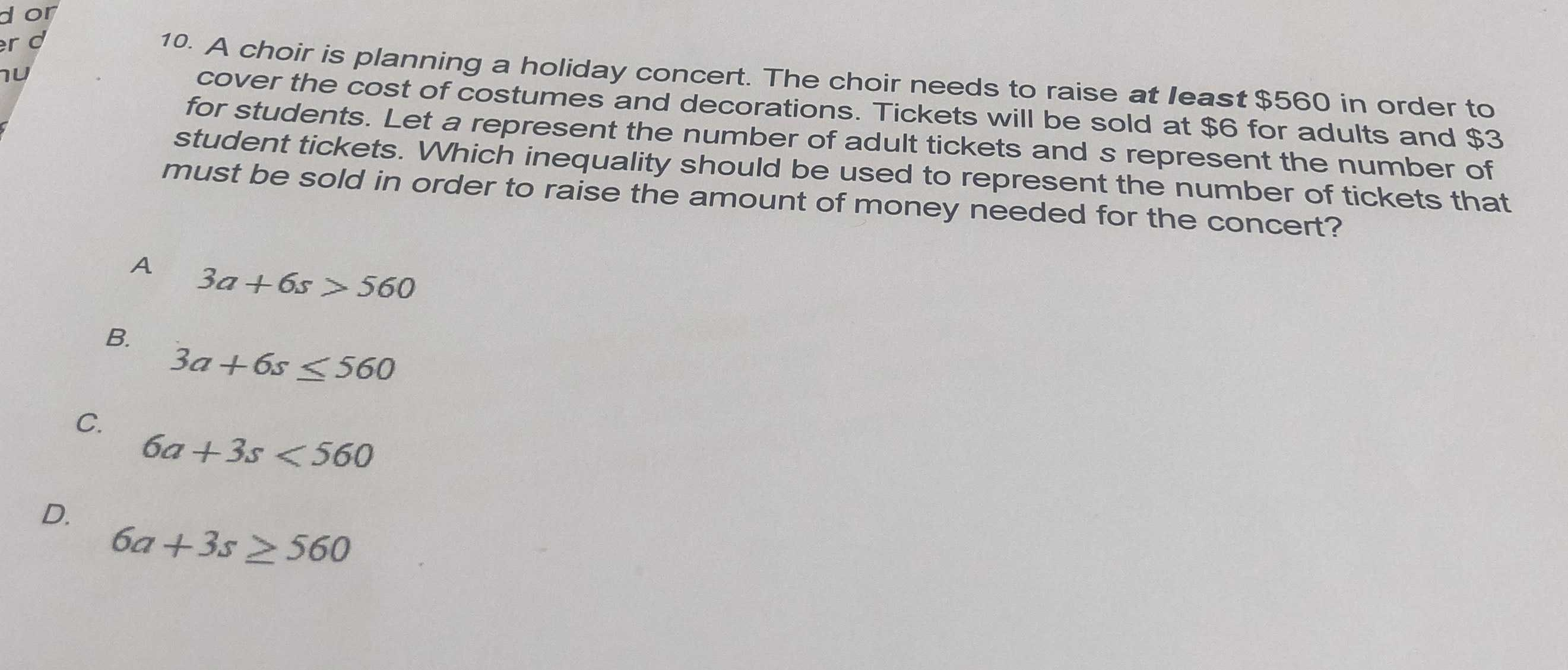### ¿Todavía tienes preguntas de matemáticas?

Pregunte a nuestros tutores expertos
Algebra
Pregunta10. A choir is planning a holiday concert. The choir needs to raise at least $$\ 560$$ in order to cover the cost of costumes and decorations. Tickets will be sold at $$\ 6$$ for adults and $$\ 3$$ for students. Let a represent the number of adult tickets and s represent the number of student tickets. Which inequality should be used to represent the number of tickets that must be sold in order to raise the amount of money needed for the concert?

A. $$3 a + 6 s > 560$$

B. $$3 a + 6 s \leq 560$$

C. $$6 a + 3 s < 560$$

D. $$6 a + 3 s \geq 560$$